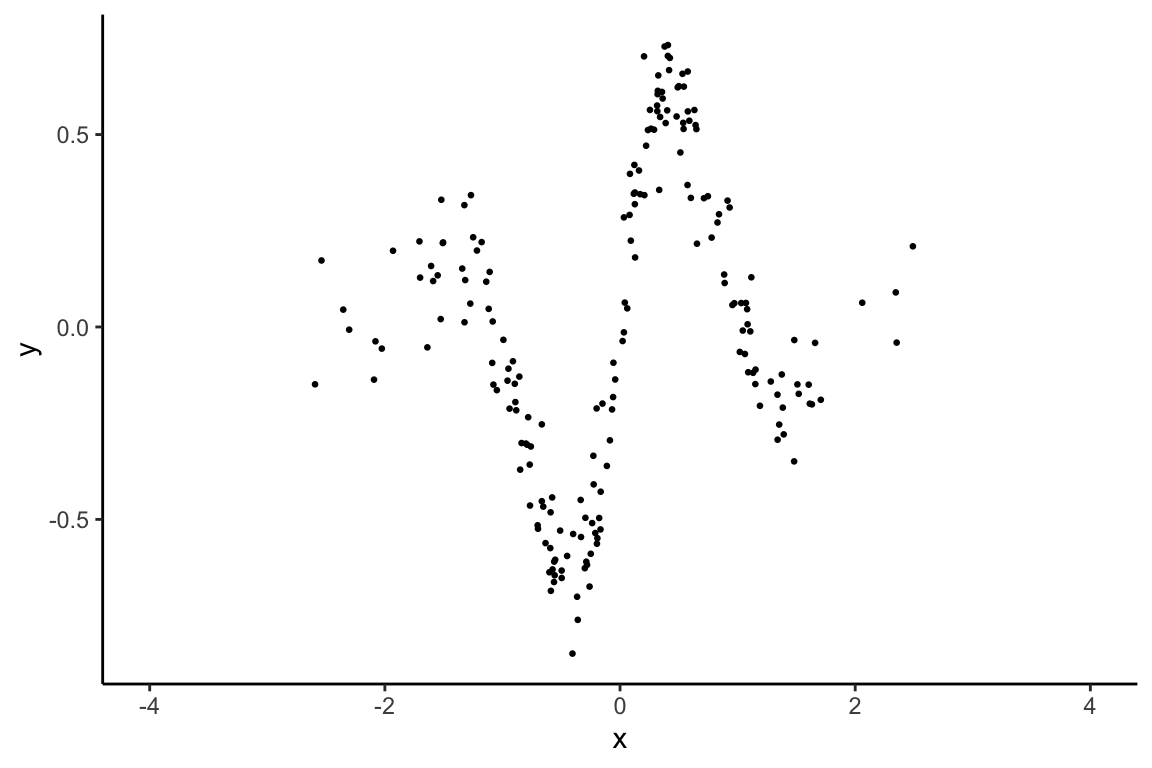This vignette shows how to use the basic functionalities of the `gplite` package. We’ll start with a simple regression example, and then illustrate the usage with non-Gaussian likelihoods. This vignette assumes that you’re already familiar Gaussian processes (GPs), and so we shall not focus on the mathematical details. To read more about GPs, see Rasmussen and Williams (2006).

# 1 Regression

``````library(gplite)
library(ggplot2)
theme_set(theme_classic())``````

Let us simulate some toy regression data.

``````# create some toy 1d regression data
set.seed(32004)
n <- 200
sigma <- 0.1
x <- rnorm(n)
y <- sin(3*x)*exp(-abs(x)) +  rnorm(n)*sigma
ggplot() +
geom_point(aes(x=x,y=y), size=0.5) +
xlab('x') + ylab('y') + xlim(-4,4)``````Set up the model with squared exponential covariance function and Gaussian likelihood. With a Gaussian observation model, we can compute the posterior analytically for a given set of hyperparameters.

So let’s first create he model. We’ll use the squared exponential covariance function for this example.

``gp <- gp_init(cfs = cf_sexp(), lik = lik_gaussian())``

There’s three hyperparameters in the model. We can see the summary of the model by printing it.

``print(gp)``
``````## Likelihood:
##    lik_gaussian(sigma = 0.5)
## Covariance functions:
##    cf_sexp(lscale = 0.3; magn = 1)
## Method:
##    method_full
## Latent approximation:
##    approx_laplace``````

We can optimize the hyperparameters to the maximum marginal likelihood solution using `gp_optim`.

``gp <- gp_optim(gp, x, y)``

Let’s print out the model and the hyperparameters after optimization:

``print(gp)``
``````## Likelihood:
##    lik_gaussian(sigma = 0.098)
## Covariance functions:
##    cf_sexp(lscale = 0.424; magn = 0.332)
## Method:
##    method_full
## Latent approximation:
##    approx_laplace``````

We can easily make predictions with the fitted model using function `gp_pred`. So let’s predict and visualize the results

``````# compute the predictive mean and variance in a grid of points
xt <- seq(-4,4,len=150)
pred <- gp_pred(gp, xt, var=T)

# visualize
mu <- pred\$mean
lb <- pred\$mean - 2*sqrt(pred\$var)
ub <- pred\$mean + 2*sqrt(pred\$var)
ggplot() +
geom_ribbon(aes(x=xt, ymin=lb, ymax=ub), fill='lightgray') +
geom_line(aes(x=xt, y=mu), size=1) +
geom_point(aes(x=x, y=y), size=0.5) +
xlab('x') + ylab('y')``````